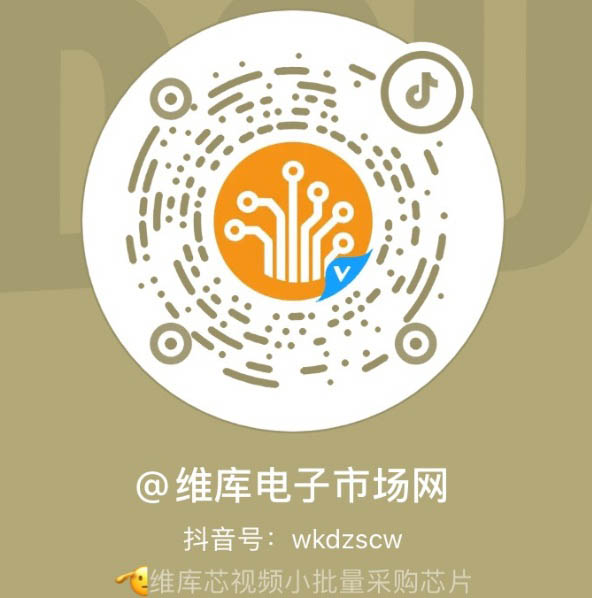• 手机端手机版芯视频 APP微信公众号维库官方抖音
• |

# 串联和并联电阻器R 2  + R 3  = 8Ω + 4Ω = 12ΩR (ab) = R comb  + R 1  = 6Ω + 6Ω = 12ΩV (R1) = I*R 1 = 1*6 = 6 伏特

V (RA) = V R4 = (12 – V R1 ) = 6 伏

I 1 = 6V ÷ R A = 6 ÷ 12 = 0.5A 或 500mA

I 2 = 6V ÷ R 4 = 6 ÷ 12 = 0.5A 或 500mA

## 电阻串联和并联示例 No2R A与R 7串联，因此总电阻将为R A + R 7 = 4 + 8 = 12Ω，如图所示。R B与R 5串联，因此总电阻将为R B + R 5 = 4 + 4 = 8Ω，如图所示。R C与R 3串联，因此总电阻将为R C + R 3 = 8Ω，如图所示。R D与R 1串联，因此总电阻将为R D + R 1 = 4 + 6 = 10Ω，如图所示。然后，上面由十个串联和并联组合连接在一起的独立电阻组成的复杂组合电阻网络可以仅用一个值为10Ω的等效电阻 ( R EQ )代替 。

### 版权与免责声明0571-85317607

9:00-17:30>>